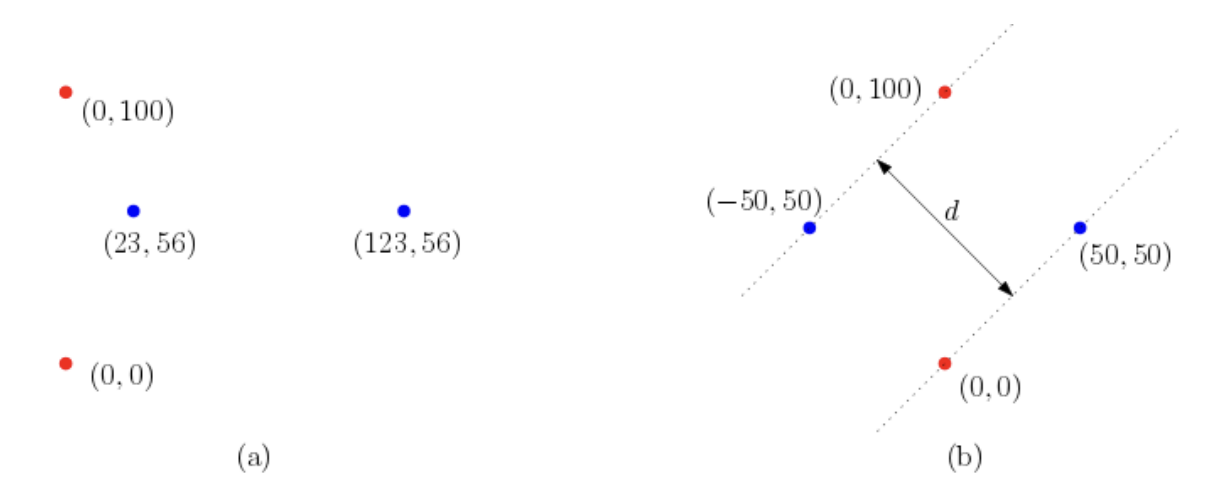시간 제한메모리 제한제출정답맞힌 사람정답 비율
1 초 512 MB0000.000%

## 문제

In the geometric matching problem, two geometric objects A and B are given, and the goal is to find an optimal transformation for B such that the transformed copy of B is as close to A as possible. Usually, the distance between A and a transformed copy of B is measured by a prescribed distance function, and one wants to minimize it over all possible transformations.

Here, we consider a simple variant of the geometric matching problem. Specifically, we assume that two input objects A and B are finite sets of points in the plane and allowed transformations for B are only translations in the plane. A translated copy of B by a two-dimensional vector v = (vx, vy) is defined to be

B + v = {(x + vx, y + vy) | (x, y) ∈ B}.

For any two-dimensional vector v, our distance function f(v) measures the smallest possible perpendicular distance between two parallel lines that contain all points of A and B + v in between. That is, we want to find an optimal two-dimensional vector v such that f(v) is minimized.Consider an example of A = {(0,0), (0,100)} and B = {(23,56), (123,56)}, depicted in the above figure (a) in which the points in A are colored red while those in B are blue. Then, consider a specific vector v = (−73, −6). The above figure (b) shows A and B + v, and two parallel lines whose perpendicular distance is d, which is exactly d = 50√2. One can verify that these two parallel lines contain all points of A and B + v in between and have the smallest possible perpendicular distance. Hence, we have f(v) = d. Further, this is the minimum possible value of f(v) over all two-dimensional vectors. Therefore, v = (−73, −6) is an optimal translation vector such that f(v) is minimized.

Given two sets of points in the plane, A and B, write a program that finds an optimal translation vector v for B such that f(v) is minimized and outputs the value of f(v).

## 입력

Your program is to read from standard input. The input starts with a line containing two integers, n (1 ≤ n ≤ 200,000) and m (1 ≤ m ≤ 200,000), where n is the number of points in the set A and m is the number of points in the set B. In each of the following n lines, the coordinates of each point in A are given by two integers separated by a space. Again, in each of the following m lines, the coordinates of each point in B are given by two integers separated by a space. The coordinates of all points given in the input range from −106 to 106, inclusively. Note that multiple points with the same coordinates can be given in each of A and B.

## 출력

Your program is to write to standard output. Print exactly one line which contains a real number z that represents the value of f(v) for an optimal two-dimensional vector v such that f(v) is minimized. The output z should be in the format that consists of its integer part, a decimal point, and its fractional part, and should satisfy the condition that f(v) − 10−6 < z < f(v) + 10−6.

## 예제 입력 1

2 2
0 0
0 100
23 56
123 56


## 예제 출력 1

70.710678


## 예제 입력 2

7 5
0 0
99 0
47 31
36 4
10 2
71 5
45 17
98 97
89 96
101 96
132 113
122 110


## 예제 출력 2

31.000000